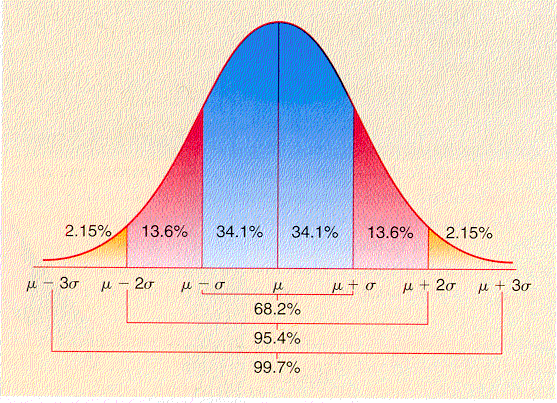## Friday, August 24, 2012

### An introduction to value at riskFrom time to time, Jurisdynamics and the other blogs in its eponymous network do offer each other positive feedback — not in the sense of "effusive praise," but in the sense of mutually reinforcing information. This is one such instance.

MoneyLaw recently announced my latest manuscript, Scholarships at Risk: The Mathematics of Merit Stipulations in Law School Financial Aid. Among other items in that paper is a brief introduction to value-at-risk (VaR) analysis. VaR is known to be flawed. It also happens to be the risk management tool of choice for investment banks and their regulators around the world. It pays to master VaR.

The following discussion of VaR, excerpted from Scholarships at Risk, draws heavily on Linda Allen et al., Understanding Market, Credit, and Operational Risk: The Value at Risk Approach (2004) and Philippe Jorion, Value at Risk: The New Benchmark for Managing Financial Risk (3d ed. 2006). I offer it as the first step toward understanding VaR.

Suppose that an investor stakes \$1 million on an index fund tracking the Standard & Poor’s 500. She asks her financial advisor, “If capital markets go down to an extent witnessed only once in a hundred trading days, what can I lose by tomorrow’s market close?”In its simplest form, VaR analysis assumes normally distributed returns. VaR1% is this quantitative tool’s answer to the investor’s question. An advisor using conventional VaR analysis may assume mean daily return of 0, with a standard deviation over that interval of 100 basis points (equal to 1 percent). On those assumptions, that advisor will report a one-day value of VaR1% as \$23,260 for a \$1 million portfolio. VaR1% = \$23,260 is a fancy, technocratic way of telling this investor that she faces a 1 percent chance of losing \$23,260 or more on her S&P 500 index fund on any given trading day. Alternatively, we could describe our investor's portfolio as having a 95 percent chance tomorrow of being worth at least \$976,740 (\$1,000,000 − \$23,260).

Using these assumptions, we shall walk through a simplified VaR analysis. Recall that we have assumed our investor has staked \$1 million in an S&P 500 index fund, where mean daily return (μ) is 0 and the standard deviation of that mean return (σ) is 100 bps (.01). The variable VaRp expresses the value at risk given a particular probability of a loss as the product of −zp, standard deviation (σ), and the total value of the portfolio (v):

VaRp = −zp · σ · v

The negative sign before −zp allows us to state value at risk as a positive sum at risk of loss. For σ = 100 bps and v = \$1,000,000:

VaRp = −zp · .01 · \$1,000,000

All that stands between us and a complete calculation of VaR1% is the value of z1%. zp is the conventional way, at least within VaR analysis, of expressing the quantile function — or the inverse cumulative distribution function — for a particular probability p. That value in turn requires the application of the quantile function. Formally:

zp = Φ−1(p) = √2 · erf−1 · (2p − 1)

Where erf−1 refers to the inverse error function.

We can now finish our worked example of elementary VaR analysis:

z.01 = Φ−1(.01) = √2 · erf−1 · (2 · .01 − 1) ≈ −2.326

Inserting this value of z1% into the formula for VaR1% yields the conclusion that VaR1% ≈ \$23,260.